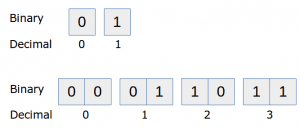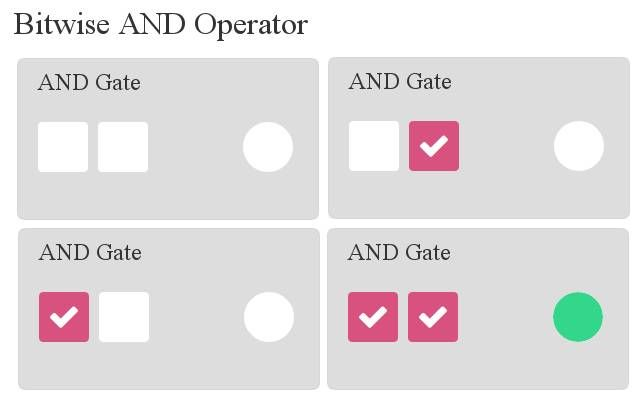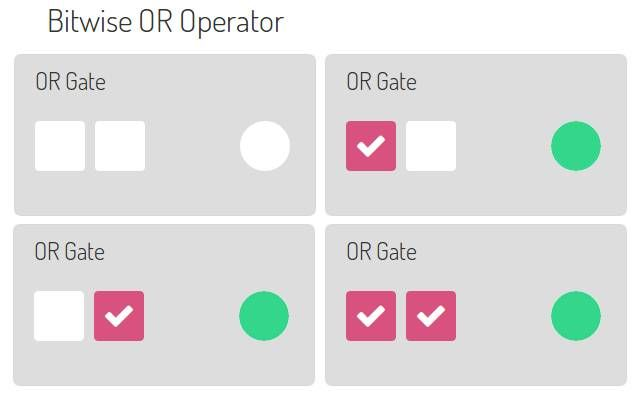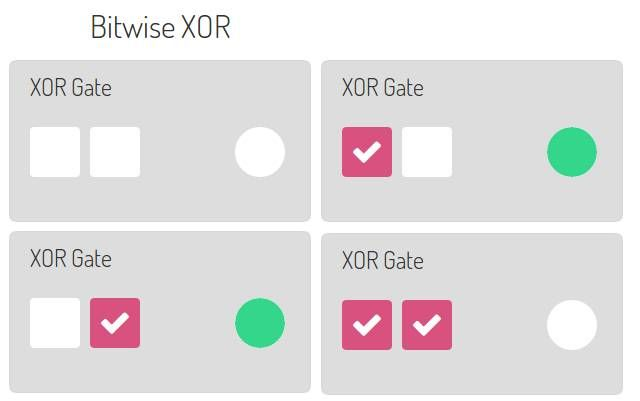# Binary numbers and logical operatorsWe have looked at simple numbers and operations before. In this article you will learn how numbers work inside the computer and a some of magic to go along with that :-)

More detailed: While this is not directly useful in web applications or most desktop applications, it is very useful to know.

In this article you will learn how to use binary numbers in Python, how to convert them to decimals and how to do bitwise operations on them.

Related course:
Python Programming Bootcamp: Go from zero to hero

## Binary numbers

At the lowest level, the computer has no notion whatsoever of numbers except ‘there is a signal’ or ‘these is not a signal’. You can think of this as a light switch: Either the switch is on or it is off.

This tiny amount of information, the smallest amount of information that you can store in a computer, is known as a bit. We represent a bit as either low (0) or high (1).

To represent higher numbers than 1, the idea was born to use a sequence of bits. A sequence of eight bits could store much larger numbers, this is called a byte. A sequence consisting of ones and zeroes is known as binary. Our traditional counting system with ten digits is known as decimal.

<caption id=”attachment_824” align=”alignnone” width=”300”]Binary numbers and their decimal representation.

Lets see that in practice:

The second parameter 2, tells Python we have a number based on 2 elements (1 and 0). To convert a byte (8 bits) to decimal, simple write a combination of eight bits in the first parameter.

How does the computer do this? Every digit (from right to left) is multiplied by the power of two.

The number ‘00010001‘ is (1 x 2^0) + (0 x 2^1) + (0 x 2^2) + (0 x 2^3) + (1 x 2^4) + (0 x 2^5) + (0 x 2^6) + (0 x 2^7) = 16 + 1 = 17. Remember, read from right to left.

The number ‘00110010’ would be (0 x 2^0) + (1 x 2^1) + (0 x 2^2) + (0 x 2^3) + (1 x 2^4) + (1 x 2^5) + (0 x 2^6) + (0 x 2^7) = 32+16+2 = 50.

Try the sequence ‘00101010’ yourself to see if you understand and verify with a Python program.

## Logical operations with binary numbers

Binary Left Shift and Binary Right Shift
Multiplication by a factor two and division by a factor of two is very easy in binary. We simply shift the bits left or right. We shift left below:

Bit 4 Bit 3 Bit 2 Bit 1
0 1 0 1
1 0 1 0

Before shifting (0,1,0,1) we have the number 5 . After shifting (1,0,1,0) we have the number 10. In python you can use the bitwise left operator (<<) to shift left and the bitwise right operator (>>) to shift right.

Output:

## The AND operator

Given two inputs, the computer can do several logic operations with those bits. Let’s take the AND operator. If input A and input B are positive, the output will be positive. We will demonstrate the AND operator graphically, the two left ones are input A and input B, the right circle is the output:

<caption id=”attachment_843” align=”alignnone” width=”640”]Bitwise AND

In code this is as simple as using the & symbol, which represents the Logical AND operator.

By changing the inputs you will have the same results as the image above. We can do the AND operator on a sequence:

Output:

This makes sense because if you do the operation by hand:

## The OR operator

Now that you have learned the AND operator, let’s have a look at the OR operator. Given two inputs, the output will be zero only if A and B are both zero.

<caption id=”attachment_847” align=”alignnone” width=”640”]binary bitwise OR

To execute it, we use the | operator. A sequence of bits can simply be executed like this:

Output:

## The XOR operator

This is an interesting operator: The Exclusive OR or shortly XOR.

<caption id=”attachment_849” align=”alignnone” width=”640”]bitwise XOR

To execute it, we use the ^ operator. A sequence of bits can simply be executed like this:

Output:

## 7 thoughts on “Binary numbers and logical operators”

1.- February 20, 2016

How to do a logical NOT operation using this way?

1.- February 22, 2016

You could use the ~ operator if you want the complements. An overview of the operators is here: https://wiki.python.org/moin/BitwiseOperators

2.- August 29, 2016

print “After shifting in binary: ” + bin(inputA << 1)

Did you know the << is getting displayed in HTML code.

2.- June 1, 2015

why is this happening???
>>> A = int(‘00100011’,2)
>>> B = int(‘00101101’,2)
>>> print bin(A & B)
0b100001
>>> print bin(A and B)
0b101101

1.- June 1, 2015

They are different operators, in the same way that * and + are different operators. The first one compares the bits, the second one compares both statements and should be used as if (a and b) then; In detail:

The first is a bitwise logical and. It compares the bits, if both are 1 it will be 1, otherwise 0. This means the computer simply looks if there is ‘high’ on both numbers at the same position.
``` 0b100011 0b101101 ------------- 0b100001 ```

The second is not a bitwise logical and operator and simply the normal and operator. If A and B then true.
I hope I explained well, if you have any questions feel free to ask. Hope you enjoy my site 🙂

1.- July 22, 2015

wish they made a quick and to the point tutorial for all langauges as you have done.

1.- October 16, 2015

Agreed. These tuts are amazing. They are concise, yet packed full of vital information. This is the only tutorial series I’ve found that is either A) able to go into bitwise operation or B) done it well at all.